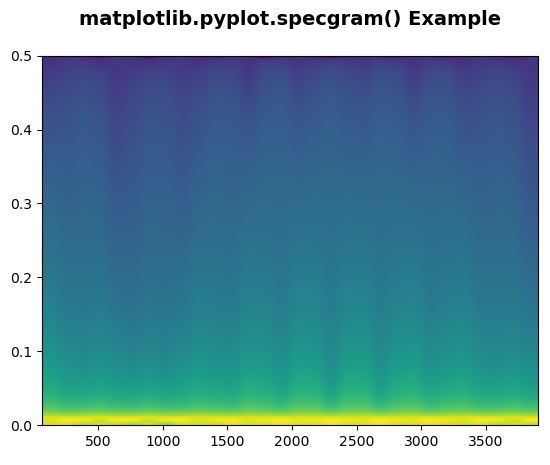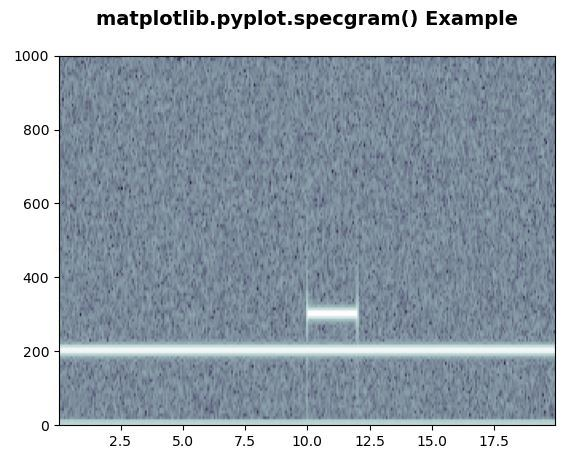# Matplotlib.pyplot.specgram() in Python

• Last Updated : 21 Apr, 2020

Matplotlib is a library in Python and it is numerical – mathematical extension for NumPy library. Pyplot is a state-based interface to a Matplotlib module which provides a MATLAB-like interface.

## matplotlib.pyplot.specgram() Function

Attention geek! Strengthen your foundations with the Python Programming Foundation Course and learn the basics.

To begin with, your interview preparations Enhance your Data Structures concepts with the Python DS Course. And to begin with your Machine Learning Journey, join the Machine Learning - Basic Level Course

The specgram() function in pyplot module of matplotlib library is used to plot a spectrogram.

Syntax: matplotlib.pyplot.specgram(x, NFFT=None, Fs=None, Fc=None, detrend=None, window=None, noverlap=None, cmap=None, xextent=None, pad_to=None, sides=None, scale_by_freq=None, mode=None, scale=None, vmin=None, vmax=None, *, data=None, **kwargs)

Parameters: This method accept the following parameters that are described below:

• x: This parameter is a sequence of data.
• Fs : This parameter is a scalar. Its default value is 2.
• window: This parameter take a data segment as an argument and return the windowed version of the segment. Its default value is window_hanning()
• sides: This parameter specifies which sides of the spectrum to return. This can have following values : ‘default’, ‘onesided’ and ‘twosided’.
• pad_to : This parameter contains the integer value to which the data segment is padded.
• Fc: This parameter is also contains the integer value to offsets the x extents of the plot to reflect the frequency range. Its default value is 0
• NFFT : This parameter contains the number of data points used in each block for the FFT.
• detrend : This parameter contains the function applied to each segment before fft-ing, designed to remove the mean or linear trend {‘none’, ‘mean’, ‘linear’}.
• scale_by_freq : This parameter is allows for integration over the returned frequency values.
• mode : This parameter is that what sort of spectrum to use {‘default’, ‘psd’, ‘magnitude’, ‘angle’, ‘phase’}.
• noverlap : This parameter is the number of points of overlap between blocks.
• scale : This parameter contains the scaling of the values in the spec {‘default’, ‘linear’, ‘dB’}.
• Fc : This parameter is the center frequency of x.
• camp: This parameter is a matplotlib.colors.Colormap instance.

Returns: This returns the following:

• spectrum :This returns the angle spectrum in radians.
• freqs :This returns the frequencies corresponding to the elements in spectrum.
• t: This returns the times corresponding to midpoints of segments.
• im: This returns the image created by imshow containing the spectrogram.

The resultant is (spectrum, freqs, t, im)

Below examples illustrate the matplotlib.pyplot.specgram() function in matplotlib.pyplot:

Example 1:

 `# Implementation of matplotlib function``import` `matplotlib.pyplot as plt ``import` `numpy as np `` ` `   ` `dt ``=` `0.005``t ``=` `np.arange(``0.0``, ``20.0``, dt) ``x ``=` `np.sin(np.pi ``*` `t) ``+` `1.5` `*` `np.cos(np.pi ``*` `2``*``t) `` ` `plt.specgram(x, Fs ``=` `1``)``plt.title(``'matplotlib.pyplot.specgram() Example\n'``, ``          ``fontsize ``=` `14``, fontweight ``=``'bold'``)`` ` `plt.show()`

Output:Example 2:

 `# Implementation of matplotlib function``import` `matplotlib.pyplot as plt ``import` `numpy as np `` ` ` ` `np.random.seed(``9360801``) ``   ` `dt ``=` `0.0005``t ``=` `np.arange(``0.0``, ``20.0``, dt) ``s1 ``=` `np.sin(``4` `*` `np.pi ``*` `100` `*` `t) ``s2 ``=` `1.5` `*` `np.sin(``1.5` `*` `np.pi ``*` `400` `*` `t) ``   ` `s2[t <``=` `10``] ``=` `s2[``12` `<``=` `t] ``=` `0``   ` `nse ``=` `0.2` `*` `np.random.random(size ``=` `len``(t)) ``   ` `x ``=` `s1 ``+` `s2 ``+` `nse   ``NFFT ``=` `512` `Fs ``=` `int``(``1.0` `/` `dt)  `` ` `plt.specgram(x, Fs ``=` `Fs, cmap ``=` `plt.cm.bone) ``plt.title(``'matplotlib.pyplot.specgram() Example\n'``,``          ``fontsize ``=` `14``, fontweight ``=``'bold'``)`` ` `plt.show()`

Output:My Personal Notes arrow_drop_up